Reach Us+44 1704 335730
SIZING OF A PV/H2 HYBRID SYSTEM TO SUPPLY PEAK LOADS ON AN ISOLATED ELECTRICAL GRID and#195;and#162;and#194;and#8364;and#194;and#8220; A CASE STUDY IN CORSICA ISLAND (FRANCE)
Global Journal of Technology and Optimization
All submissions of the EM system will be redirected to Online Manuscript Submission System. Authors are requested to submit articles directly to Online Manuscript Submission System of respective journal.

# SIZING OF A PV/H2 HYBRID SYSTEM TO SUPPLY PEAK LOADS ON AN ISOLATED ELECTRICAL GRID Ã¢Â€Â“ A CASE STUDY IN CORSICA ISLAND (FRANCE)

P.Poggi*, C.Cristofari, J.I. Canaletti, C.Darras, M.Muselli

University of Corsica, UMR CNRS SPE, Route des Sanguinaires, France

*Corresponding Author:
P.Poggi
University of Corsica, UMR CNRS SPE, Route des Sanguinaires, France
E-mail: [email protected]

Received date: May 2008; Revised date: September 2008; Accepted date: March 2009

Visit for more related articles at Global Journal of Technology and Optimization

#### Abstract

The object of the study is to reduce the daily load peaks on an insular electric network (Corsica Island, France) using with help to renewable energy systems. The methodology is based on an energy balance between a PV array, an electrolyzer and fuel cells (50 kW) supplying a seasonal load profile built from electrical network load peaks. This load is supplied firstly by the PV array and the fuel cell produces complementary power when the PV system is deficient. An electrolyzer, using unconsumed PV energy produces hydrogen for the fuel cell. In the present work, at the beginning of the operation, the hydrogen stock is sufficient to satisfy a given number of days corresponding to the system autonomy (Loss of Load probability = 0). Solar and ambient temperature data were measured at our laboratory weather station in Ajaccio (41°55' N, 8°48' E, 70 m altitude). Hourly data are available for the years 1998 to 2004. The originality of this approach was to determine a whole methodology allowing obtaining quickly sizing curves for a PV/H2 hybrid system supplying a given load (remote sites and/or grid connection).

#### Keywords

Modeling, economic, PV, fuel cell

#### Introduction

The energetic hybrid system PV-electrolyzer-fuel cell has been studied since the eighties. The interest for the solution of long term storage of energy with the help of hydrogen vector grows continuously. Several scientific publications have already been published concerning different topics in relation to the coupling between H2 production and renewable energy sources. The literature  presents some recent works about projects of coupling EnR-H2 installed throughout the world:

- EOLHY project: a PEM of 2.5 kW in 2006 in France. The objective is an autonomous uninterrupted power supply starting from intermittent renewable sources by production and storage of hydrogen and fuel cell.

- UTSIRA and IFE project: respectively a PEM of 10 and 0.5 kW in 2004 and to Ife in 2002 in Norway. The objective of the project is to demonstrate an autonomous energy system. To integrate developed hydrogen technologies with renewable energies.

- “Trois rivières” project: a PEM of 5 kW. A stand-alone renewable energy system based on hydrogen production from wind and solar energy was developed and installed at the Hydrogen Research Institute (HRI) in Canada.

The present work is associated to a research program called PEPITE accepted in September 2007 by the National Research Agency of France within the framework of the Pan-H program. The aim of the whole study is to build a computational tool able to establish sizing curves and optimized hybrid PV-H2 systems in order to supply an electrical grid or remote loads. PEPITE will allow obtaining references to optimize the later realizations of these systems and to make a success of a significant experimentation, in order to prove the feasibility of EnR-H2 electrification.

PEPITE represents the previous step for the MYRTE project corresponding to the implementation of a large PV/H2 plant in Corsica. The main objective of witch will be to reduce load peaks on the grid by using H2 storage via an hydrogen chain (electrolyzer, compressor, fuel cell). In its achieved development, MYRTE project will represent 3.6 MW of PV array, coupled with a fuel cell park of 200 kW in order to limit the load peak. In the first step of the plant construction (2008), MYRTE will use a 50 kW fuel cell.

Modeling and Simulating

Load profiles have been built from electrical power curves measured in 2005, on the isolated insular grid in Corsica by EDF (Electricité de France) organization. The mean daily energy supplied by the grid represents respectively 8557 MWh in winter, 5459 MWh in summer and 3023 MWh in autumn/spring. In term of power, the peak load in winter can reach 429 MW in winter, with a maximum of 175 MW in middle seasons and 287 MW in summer.

Reducing load peaks using renewable energy systems coupled with fuel cells corresponds to supply of 7% the energy of the peak loads. For this work, a seasonal typical load profile presents two peak demands which correspond to one peak measured around 10:00 am (± 3 hours) for all seasons with an amplitude of about 20 MW and the second one depending strongly of the yearly period: in winter, around 6:00 pm (± 2 hours) corresponding to 50 kW and around 9:00 pm (± 2 hours) in the range of 20-25 MW for others seasons (Figure 1).

Presentation of the system

A PV array, an electrolyzer, a storage tank for hydrogen, a FC and some pumps to compress hydrogen compose the system architecture (Figure 2). Hourly solar (45° tilted global) and ambient temperature profiles have been measured (standard time) from the laboratory weather station of Ajaccio (41°55' N, 8°48' E, 70 m asl). These data are available for the years 1998 to 2004. In relation to the PV plant, we implement a DC/DC converter corresponding to MPPT device (Maximum Power Point Tracker). Energy necessary to the load will be provided in priority by the PV plant using a DC/AC converter. When the production of the PV plant is insufficient, the fuel cell FC will provide the missing energy. The nominal FC power has been chosen equal to the maximum daily peak load i.e. 50 kW. The electrolyzer will be supplied when a surplus of PV energy is available. The electrolyzer nominal power is built from the nominal PV power plant (see below). A compressor allows to limit H2 volume storage using multi-stage compression (γ =6/5) at 200 bars. For the present study, we optimize the system for no supply failure, corresponding to a load in total autonomy (Loss of Load Probability LOLP = 0). For each PV nominal power (kW), the computational simulation determines the H2 storage volume (Nm3) or H2 mass (kg) corresponding to the system autonomy (i.e. no default in the load supplying).

The principal interest of this energetic balance model lies in its simplicity and its facility of use, which implies fast times of simulation.

Modeling of Each Sub-systems PV array

The model used for determining the hourly PV array energy output is the polynomial model described by the following equation , , :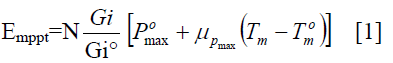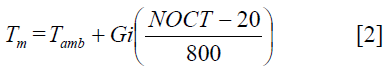Where:

N : module numbers

Gi : total solar irradiation of the considered place (Wh.m-2)

Gi° : solar irradiation under the standard conditions (1000 Wh.m-2)

Pomax : maximum power of the PV module under the standard conditions (W)

μpmax : coefficient of variation of the power according to the temperature (%/K)

Tm : operating cell temperature according to the solar irradiation and the ambient temperature (K)

Tom : PV module temperature under the standard conditions (298K)

Tamb : ambient temperature (K)

NOCT : operating temperature of the photovoltaic cells under the following conditions: a irradiation sunning of 800 W/m², an ambient temperature of 293K and an optical mass of air AM equalizes to 1 (K)

The model allows simulating any type of module statement starting from the design features data of the manufacturer. Hourly global solar radiation (45° tilted) and ambient temperature profiles were measured (local time) from the weather station of Ajaccio (41°55' N, 8°48' E, 70 m asl) in Corsica Island (France). These data are available for the years March 1998 to December 2004 (56520 hours).

DC/AC converter

The model used for determining the converter efficiency is a physic model , , .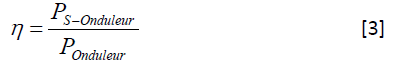with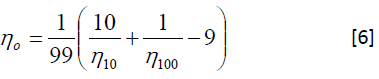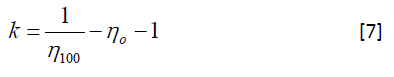where

PE Onduleur − Input power of the converter (W)

PS Onduleur − Output power of the converter (W)

ηOnduleur Converter efficiency of the converter

ηo Constant independent of the load, losses from the vacuums

k Constant from resistive losses of the converter

POnduleur Nominal power of the converter (W)

η10 Converter efficiency at 10% nominal power (manufacturer curve)

η100 Converter efficiency at 100% nominal power (manufacturer curve)

Electrolyzer

The energy produced by PV array and which is not consumed by the load, is used to feed the electrolyzer to produce hydrogen. If, during the system operation, this power falls bellow a given preset energy level, the controller commanding the electrolyzer will switch it off. This method of monitoring and triggering allows effective energy management between the input energy sources, the electrolyzer input, the fuel cell and the load.

The available energy is computed and included in the interval delimited by a high and low threshold due to the principle of operating of the electrolyzer. In order to work, this sub-system needs a minimum of energy (low threshold) and it is limited in power (high threshold). It is assumed that the electrolyzer has high response times, hence they are negligible in comparison to the time of simulation (1 hour). In order to minimize the energy losses, his nominal power must be computed as a preliminary task using the PV array size. The optimum power of the electrolyzer is sought for each PV array size: the electrolyzed energy is drawn in accordance with the electrolyzer power (Figure 3).The maximal power of the electrolyzer (high threshold) is defined as a percentage calculated for each value of the PV peak power array installed. (typically between 60 to 80%) The low threshold is defined as a percentage (typically 20%) of the minimal power of the electrolyzer .

The consumption of the compressor is determined as a percentage  of the available energy at the input of the electrolyzer. This hypothesis induces to consider that the compressor and the electrolyzer are starting at the same moment, and the consumption of the compressor depends on the electrolyzer hydrogen production. This consumption percentage of the electrolyzer (which is the energy required to produce hydrogen) is calculated by the specific energy compression given by the relation (eq.8) . The efficiency of this conversion is obtained by the equation (eq.10) :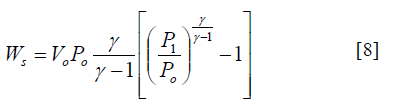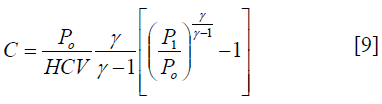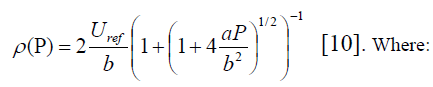Ws : compression energy (J/kg)

γ : ratio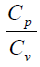(1.2 for multi-stage compression)

Po : hydrogen pressure at electrolyzer output

Vo : H2 initial specific volume (11.11 m3/kg at 1 bar)

P1 : final hydrogen pressure in the tank

C : consumption percentage of the compressor (%)

HCV : hydrogen High Calorific Value (12.78 J/Nm3)

ρ (P) : efficiency of this conversion (function of the electrical energy P at the input of the electrolyzer)

Uref : standard reference voltage (1.25V)

a and b : parameter characteristics of the electrolyzer curve (U=a.I+b)

Fuel Cell

The necessary energy used by the load which is not produced by the PV system should come from the fuel cells. The provided energy difference was computed hour by hour and low and high energy threshold were introduced. The response time was considered very fast in comparison with the hourly time-step of the simulation. The efficiency of this conversion (eq.11)  is a function of the electrical energy to be provided by the FC.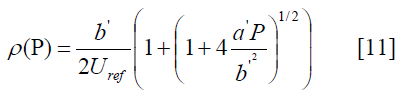Where a’ and b’ are the parameter characteristics of the fuel cell curve (U=a’I+b’)

There are two kinds of energy supply failure. The first one concerns the hydrogen storage, and occurs if the autonomy of hydrogen storage is not sufficient. The second one concerns the power default which occurs if the load reaches a non-common load peak.

Hydrogen storage

At each hour, the hydrogen flow in the tank is the difference between the hydrogen produced by the electrolyzer and the hydrogen consumed by the fuel cells to satisfy the load. The hydrogen volume in the tank during the year is the sum of these flows.

We reduce the hydrogen production to a percentage for simulate the losses of hydrogen in the system (typically 5%) . The quantity of H2 in the stock is adjusted with the days of autonomy in view to assume the load with LOLP = 0.

The total stock of hydrogen is finally compressed at a given pressure (200 bars) by the model (eq.1) :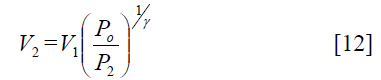where

V2 : volume compressed (m3)

V1 : initial volume (m3)

P2 : tank pressure (Pa)

#### Results

The average system consumption is 150 kWh per day, which corresponds approximately to 9 kg daily hydrogen mass. The results of the present work are based on a number of different days of autonomy (3.5, 7, and 10 days) in order to simulate short, medium and long term storage. This storage autonomy limits the H2 quantity that the system can store. The minimum H2 storage mass was computed in order to install a system which does not suffer from energy supply break down. When the number of days of autonomy increases, the quantity of hydrogen increases and necessary the number of module to be installed decreases

Figure 4 represents the optimization sizing curve for the PV/H2 system corresponding to H2 mass (kg) versus installed power of the PV array allowing to respect LOLP = 0. All the couples (MH2, Ppv) lead to a load autonomy (black line, Figure 1). It can be seen that the more PV power is installed, the more quantity of the H2 decreases.

Figure 4: Sizing curve for the given load. Each PV/H2 configuration (2D vector with a nominal PV power in kW and a storage H2 mass in kg) leads to LOLP = 0.

Figure 5 shows the energy consumed by the load (powered from the PV array or from the fuel cell) from a relative point of view (that is divided by the total energy consumed by this one), versus PV array power. We define a PV cover rate corresponding the PV energy produced allowing to supply the load during the whole simulation: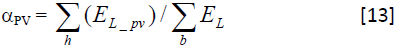where

EL_pv : PV energy produced to supply the load for each simulation hour h

EL : Load energy for the corresponding hour h

αFC : Load FC cover rate which is computed as the fuel cell energy allowing to supply the load profile (αFC + αPV = 1)

The sum of both PV and FC cover rates for any simulation time-step corresponds to 100 % of the consumption of the load. The system never falls out of order.

The PV cover rate respectively increases of 5% and 2% when days of autonomy decrease from 10 to 7 and from 7 to 3.5. The curve presents phenomenon saturation (horizontal tangent); From Ppv in the range 300-600 kW, αPV remains quasi static (only +5 %).

Figure 6 shows the relative running time for fuel cell and electrolyzer (that is divided by the time of functioning of the system), according to the power of the PV array installed. We observe that the more PV power is installed, the less fuel cell is used, and the more the electrolyzer is used to store H2.

The relative running time for fuel cell respectively increases of 2% and 3% when the days of autonomy go up from 3.5 to 7 and from 7 to 10. The relative running time for electrolyzer increases of 1% when the days of autonomy passes from 3.5 to 7 and from 7 to 10.

Figure 7(a,b,c) shows respectively the H2 quantity advancement for an installed PV power of 85, 99 and 124 kW. These powers correspond with the minimal values which to be installed so that the system does not fall broken down. The graph shows that some weather events generate an important increase in the system sizing. It should be interesting to put a system plug, a battery park, or a LOLP ≠ 0, to reduce the size of the system.

Figure 7: H2 quantity advancement for PV installed power : (a) : 124 kW (3.5 autonomy days, LOLP = 0), (b) : 99 kW (7 days, , LOLP = 0), (c) : 85kW (10 days, LOLP = 0), (d) : 87.5 kW (LOLP = 1%) instead of 99 kW (LOLP = 0%) with a H2 storage configuration allowing 7 days of autonomy.

Figure 7(d) shows the H2 quantity advancement for a power of 87.5kW PV array installed. This powers correspond to LOLP close to 1%.

Tableau 1 shows the PV array installed, the decreasing of the system sizing and the numbers of hours of break down for different LOLP.

#### Conclusion

This first PV-H2 modelization approach allows to build, with a quick simulation (a few minutes), sizing curves for PV and H2 chains in a renewable energy system. The definition of couples (Ppv, MH2), in combination with a fixed number of days of autonomy can determine the ratio between installed PV power and H2 mass required to implement a suitable loss of load probability (LOLP).

First results on the system sizing impact for LOLP ≠ 0 are presented showing that a 1% annual reduction of the load supply allows reducing the installed nominal PV power by about 12%.

An optimization procedure based on the kWh cost produced by the system is necessary to obtain the optimal configuration for each LOLP. In future works, an. improvement of the sub-system behavior (specially electrolyzer and fuel cell ageing) will allow the best description of the whole system for a better equilibrium between renewable energy production and load consumption

#### References

Select your language of interest to view the total content in your interested language

### Article Usage

• Total views: 11864
• [From(publication date):
June-2010 - Jul 16, 2019]
• Breakdown by view type
• HTML page views : 8058Can't read the image? click here to refresh